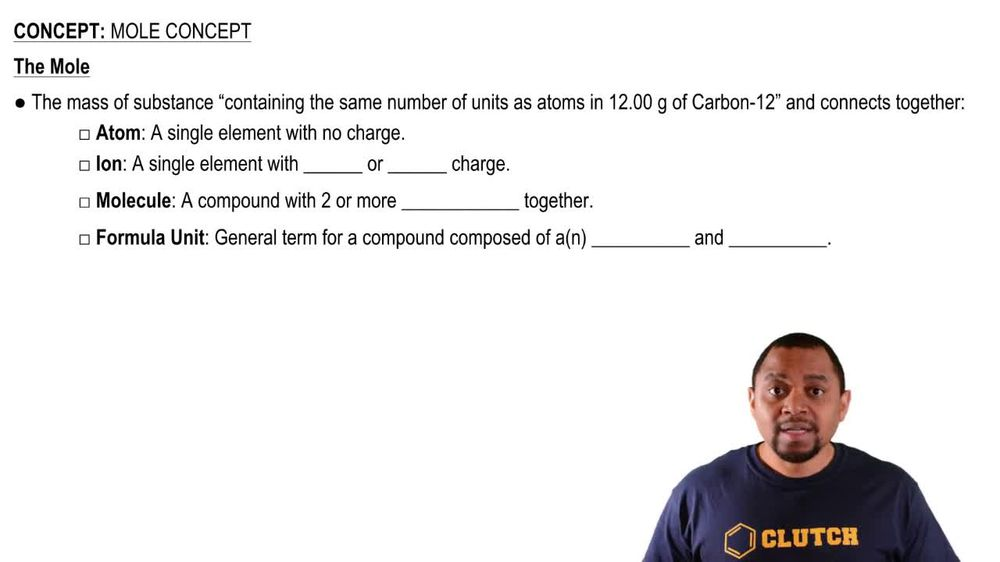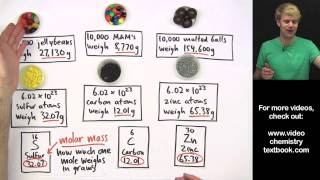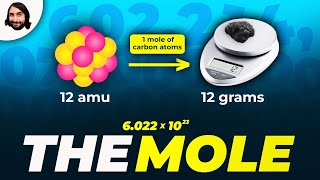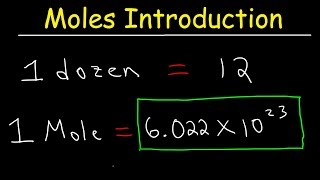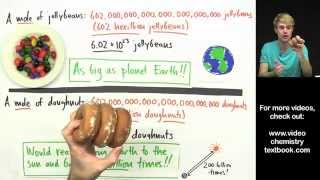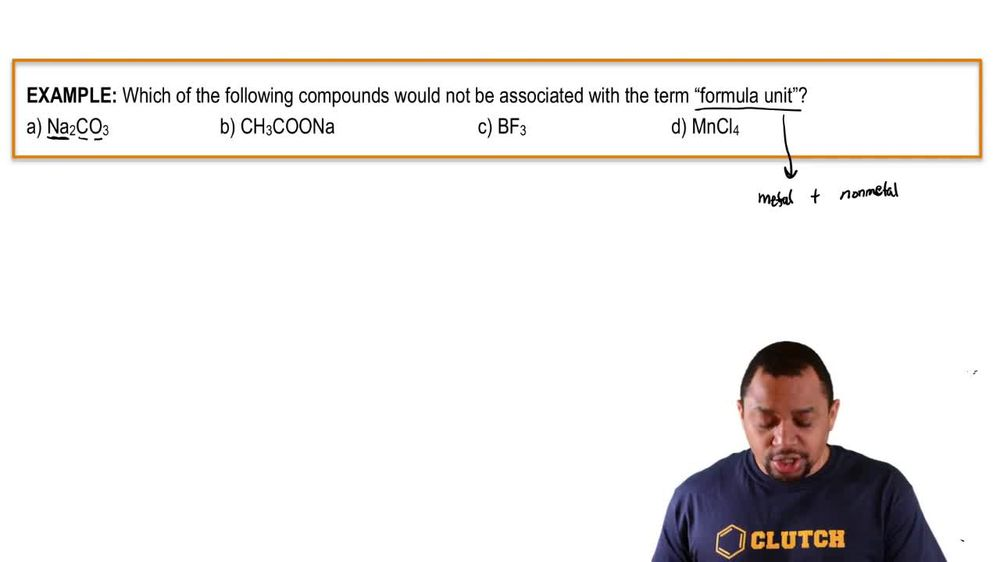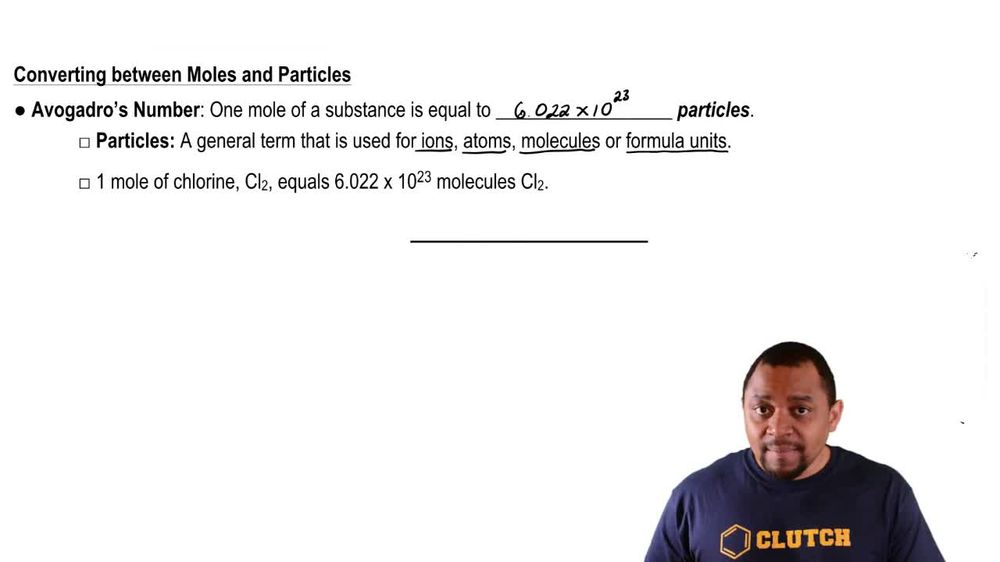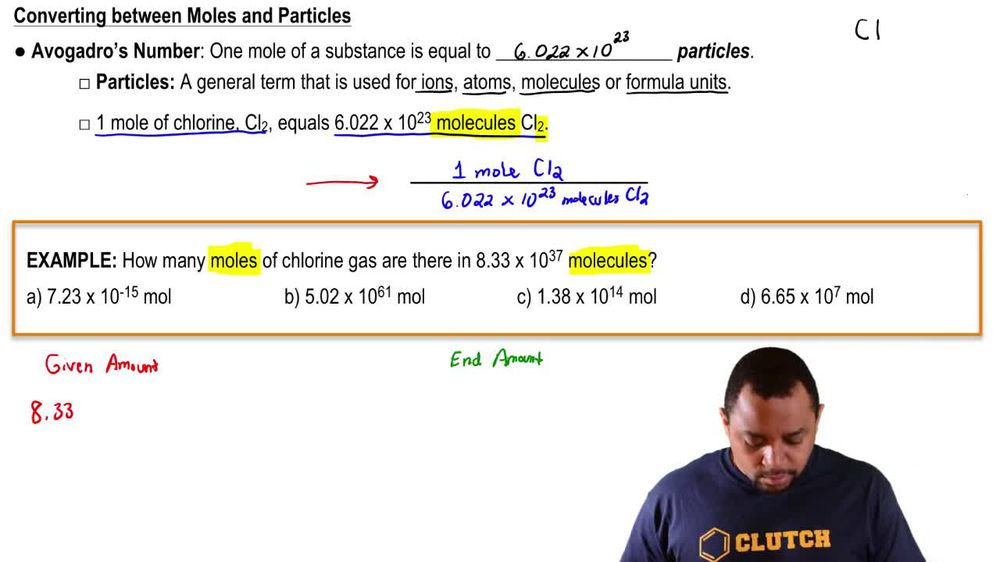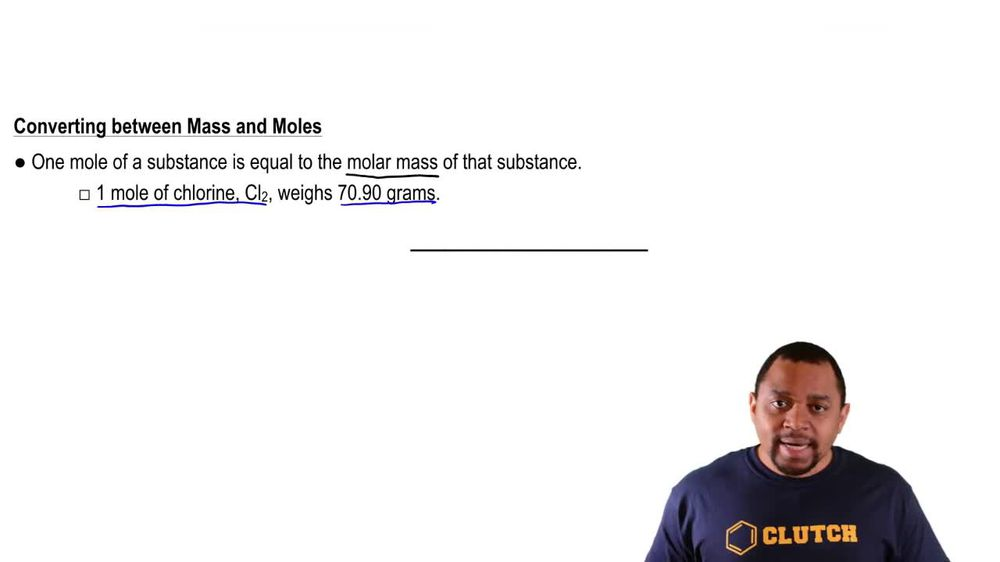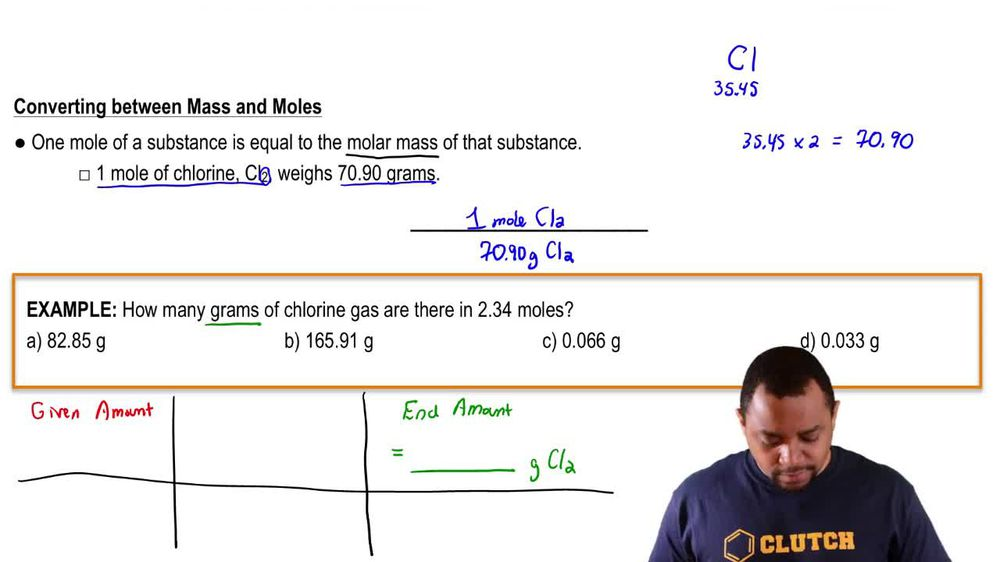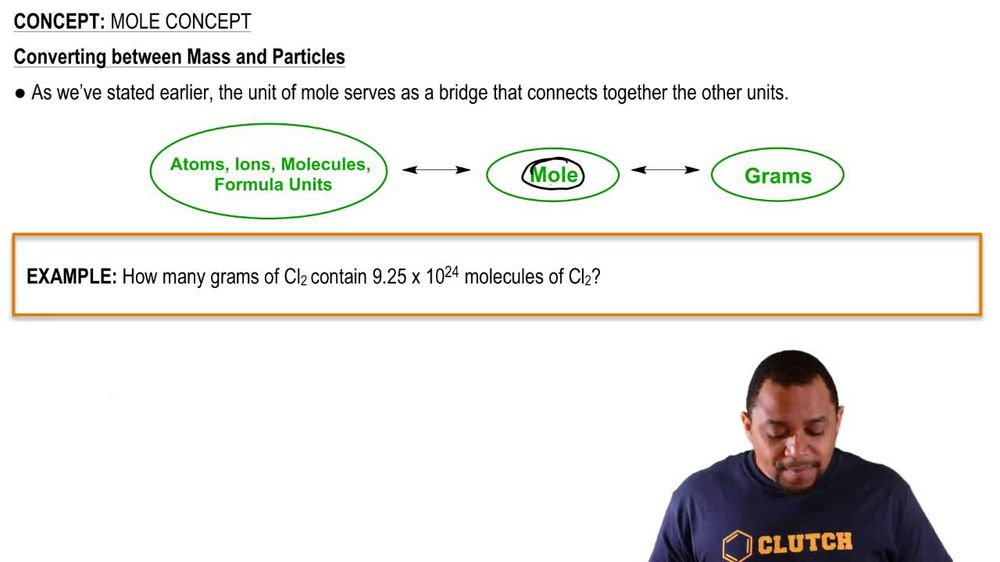Start typing, then use the up and down arrows to select an option from the list.
1. 2. Atoms & Elements2. Mole Concept
Problem

# Calculate the mass (in g) of each sample. b. 9.85 * 1019 CCl2F2 molecules

Relevant Solution1m
Play a video:
Hello everyone in this question we asked calculate the mass in kilograms Of 8.63 times 10 to the 26 molecules of carbon di sulfide. Given that the Mueller Masters 76.14 g per mole, They were given 8.63 I'm center molecules of carbon di sulfide. And we need to first convert to moles using avocados number, Which is 6.0-2 Times 10 to molecules of carbon di sulfide in one mole of carbon and sulfide. And now we can convert to grams using the molar mass oh and one more of carbon and sulfide Whatever, Mueller Mast which is 76.14 programs. And now we can convert from grams to kilograms. We have 1000 g in one kg And this will give us 109 0.1 telegrams, which is deep. Thanks for watching my video and I hope it was helpful.